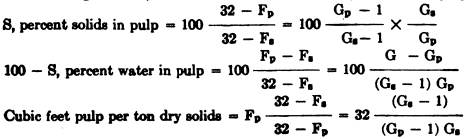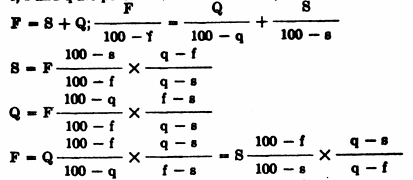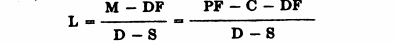# Liquid-Solid Relationships Specific Gravity Specific Volume

### Gravity Specific Volume

Practically all metals occur as minerals in the earth’s crust. One of the most important characteristics of minerals is the relative weight, usually measured as specific gravity.

The specific gravity of a solid or liquid is the ratio of its Weight to the weight of an equal volume of water under standard conditions. Density is any weight/volume relationship, including specific gravity. The specific gravity of water is 1.000 at 4° C. WATER at maximum density, 4° C., 39° F.

• 1 cu. ft. weighs 62.4283 lbs. = 28.317 kilogram. 1 cu. in. = 0.578 os.
• 1 cu. centimeter (cc) weighs 1 gram.
• 1 ton equals 32.04 cu. ft. under above conditions.
• 1 ton equals 32.2 cu. ft. at 90° F.

The above value will be reduced by impurities always present in ordinary tap water, so that a value of 32 cu. ft. per ton (32 C.F.P.T.) may be used in ordinary mill calculations.

Sea water contains in solution about 3.44% soluble salts (of which 2.5 is common salt), weighs 64.176 lbs. per cubic foot, average 31.13 C.F.P.T. and 1.028 specific gravity. The concentrated waters in Great Salt Lake and the Dead Sea contain about 22.4% soluble salts, weigh 73.5 lbs. per cubic foot, average 27.2 C.F.P.T., and 1.17 specific gravity.

The reciprocal of specific gravity is specific volume. A more convenient form of specific volume for use in mill calculations is cubic-feet-per-ton (C.F.P.T.);

specific gravity = 32/ C.F.P.T.  and C.F.P.T. = 32/ SG

Determination of specific gravity and CFPT: Solids; weigh up 32 grams of dry solids in small enough pieces to pour into a 50 cc. glass graduate; fill the latter to a definite mark, 25 cc. or 30 cc.; pour in the solids; the rise in cc. is C.F.P.T., and-is the 32/C.F.P.T. specific gravity. For more accurate work use 320 grams and a 500 cc. graduate, and divide rise by 10 for C.F.P.T.

This method may be used for pulps, except for the sticking of solids to the side of the graduate; the usual method is with a SG flask. Fill the flask to the mark, weigh it, deduct the empty weight of flask, and divide the net weight in grams by the volume in cc., which gives SG.

The following relations exist between the constituents of a mixture which contains no voids, such as a pulp, or a rock.by volume where a and b are fractional parts (usually totalling 100%) by weight; A and B are fractional parts by volume; Fa, Fb and Fe are C.F.P.T. of the two substances and their combinations; Ga, Gb and Gc are specific gravities of the two substances and their combination.

The following formulae, derived from the fundamentals, are useful in pulp calculations:F and G refer to C.F.P.T. and SG; subscripts p and s to pulps and solids. Similar formula applied to moisture values are useful for calculations on circulating loads from classifiers, and for thickeners, particularly for operations:

F, 8, and Q are dry tons of feed, sands and overflow.

f, s and q are percent of moisture in feed, sands and overflow.Five quantities must be known to find the other. The following method of calculating the circulating load in closed circuited grinding is from F. C. Bond, A.I.M.E., Mining A Metallurgy, November 1937:Where L is dry tons per hour of classifier returned Sand.

• F is dry tons per hour of new feed to mill.
• D is dilution (parts liquid to 1 part solids) mill Discharge.
• 8 is dilution (parts liquid to 1 part solids) classifier 8and.
• P is dilution (parts liquid to 1 part solids) classifier Overflow.
• C is tons per hour, new water added to classifier.
• M is tons per hour, new water added to mill feed, including moisture in ore. The circulating load relationship is expressed also by the following:

Q = 100T / (100 – P)

Where: